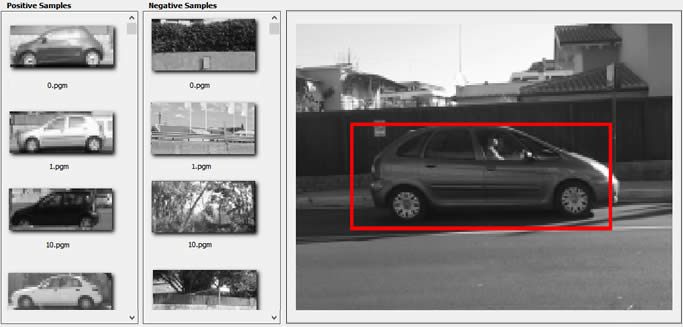ImageEn, unit ievision

#### Declaration

procedure createSamples(imagename: PAnsiChar; bgImagesDir: PAnsiChar; vecname: PAnsiChar; num: int32_t = 1000; width: int32_t = 24; height: int32_t = 24; bgcolor: int32_t = 0; bgthreshold: int32_t = 80; inv: bool32 = false; randomInvert: bool32 = false; maxintensitydev: int32_t = 40; maxxangle: double = 1.1; maxyangle: double = 1.1; maxzangle: double = 0.5; infoname: PAnsiChar = nil; maxscale = -1.0); safecall;

#### Description

Create a file containing a set of positive samples, generated from a single positive image.
The result of this function (named "vec" file) is then passed to trainCascade for the actual training.
If you have a set of real positive images please use createSamplesFromImageSet to create the file of positive samples.Parameter Description
imagename Source object image (the single positive sample)
bgImagesDir Path to a directory containing a list of images which are used as a background for randomly distorted versions of the object
vecname Name of the output file containing the positive samples for training
num Number of positive samples to generate
width Width (in pixels) of the output samples
height Height (in pixels) of the output samples
bgcolor Background color (0..255, as gray scale value). The background color denotes the transparent color
bgthreshold Background color tolerance. All pixels withing bgcolor-bgthresh and bgcolor+bgthresh range are interpreted as transparent
inv If True colors will be inverted
randomInvert If True colors will be inverted randomly
maxintensitydev Maximal intensity deviation of pixels in foreground samples
maxxangle Maximal rotation angle towards x-axis, must be given in radians
maxyangle Maximal rotation angle towards y-axis, must be given in radians
maxzangle Maximal rotation angle towards z-axis, must be given in radians
infoname Use an alternative way to create output file from a list of samples. "infoname" specifies a text file containing following information for each row: filepath, number of objects in this image
"x y width height" for each object. All values separated by spaces. A row for each image
maxscale Max sample scale